# Decimal Math Games

Are you looking for free decimal math games? On this page you can find games for the following important topics: rounding decimals to the nearest whole number, estimating sums with decimals, comparing and ordering decimals, adding and subtracting decimals using tenths, hundredths, and thousandths, multiplying decimals, multiplying decimals with whole numbers, changing decimals to fractions and percents, and solving word problems with decimals.

The math games on this page are designed to reinforce basic facts about decimals. Students all over the world love soccer. They will probably love these math soccer games about decimals.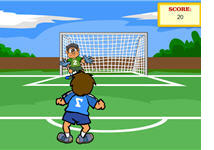Adding Decimals
Add tenths, hundredths, and thousandths with sums greater than one.

Subtracting Decimals Soccer
Fun soccer game about subtracting decimals.

Rounding Decimals to the Nearest Whole Number
Learn how to round decimals by playing this exciting game.

How many homeruns can you hit? Play the following fast-paced baseball math games about decimals to show off your skills.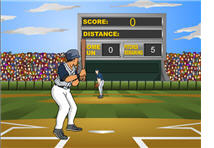Rounding Decimals
Students can practice rounding decimals to different place values.

Add decimals and earn hundreds of points when playing this fun baseball game.

Subtracting Decimals Baseball
Subtract decimals in this fast-paced baseball math game.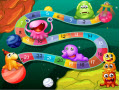Decimals Board Game

Check your knowledge with this online decimals board game and be the first one to get to the finish line. For each correct answer, you will be able to roll the die to move forward on the game board to get to the finish line.

Do you love football? Try playing these fun football math games about decimals.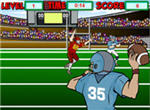Decimals Place Value
Solve problems about place value in decimals in this interactive football math game.

Play the following basketball math games alone or with a partner. These exciting games can also be played in the classroom by using a Promethean Board or an LCD projector and dividing the students into two teams.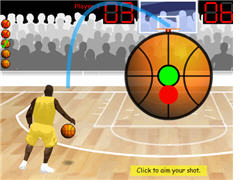Adding Decimals Game

Subtract decimals correctly to score as many points as possible.

Multiplying Decimals Game
Do yo know how to multiply decimals? Show off your knowledge and skills by playing this game.

The following jeopardy games have a single-player mode, as well as a multi-player feature. You can play these games at home or at school as review activities.Decimals Jeopardy Game
Review the four operations with decimals by playing this jeopardy game.

Fractions, Decimals, Percets Jeopardy Game
Change fractions to decimals and percents and vice-versa.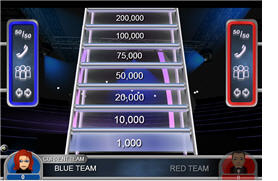Changing Fractions and Decimals to Percents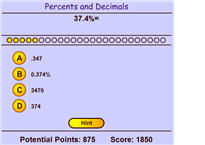Demonstrate your math skills and earn points by playing this interactive game about changing decimals to percents and vice-versa.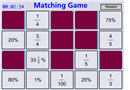Changing Fractions to Percents
In this concentration game, students will match different fractions with the equivalent percents.

Return from the Decimal Math Games page to the Math Play homepage.